Function Repository Resource:

Compute the polar radius of a regular polygon

Contributed by: Erik Mahieu
 ResourceFunction["RegularPolygonAngleRadius"][t,n] computes the polar radius at angle t with respect to the x axis of a regular polygon with n vertices equally spaced around the unit circle. ResourceFunction["RegularPolygonAngleRadius"][t,r,n] computes the radius of a regular polygon whose vertices lie on a circle of radius r. ResourceFunction["RegularPolygonAngleRadius"][t,t0,r,n] starts at angle t0 with respect to the x axis.

## Examples

### Basic Examples (1)

Draw the perimeter of a regular polygon using polar coordinates:

 In:=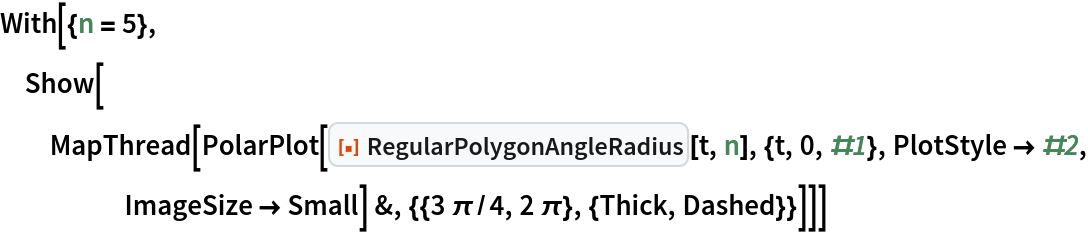Out=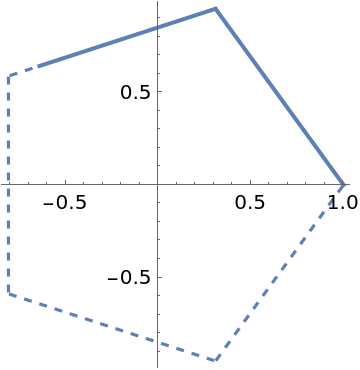### Scope (2)

Use a different radius and starting angle:

 In:=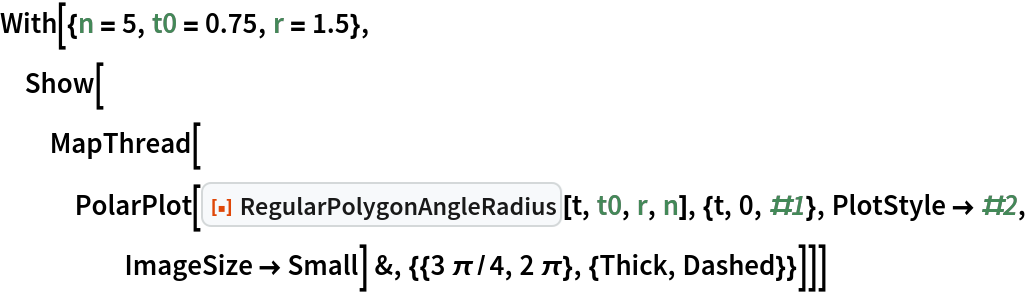Out=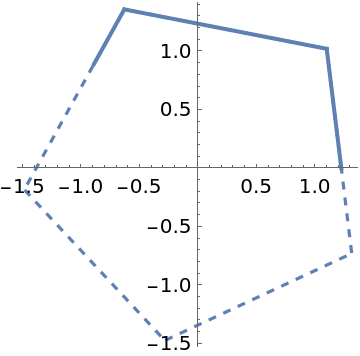Draw the perimeter of any regular polygon using parametric coordinates:

 In:=Out=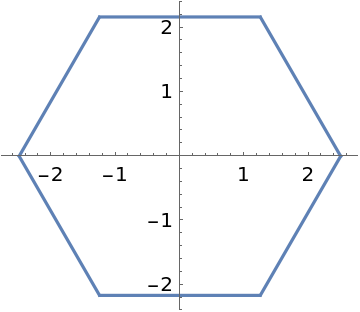### Applications (1)

Construct a cylinder with a polygonal cross-section:

 In:=Out=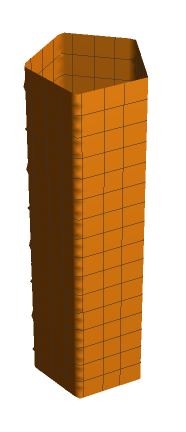### Properties and Relations (2)

RegularPolygonAngleRadius gives the boundary of RegularPolygon:

 In:=Out=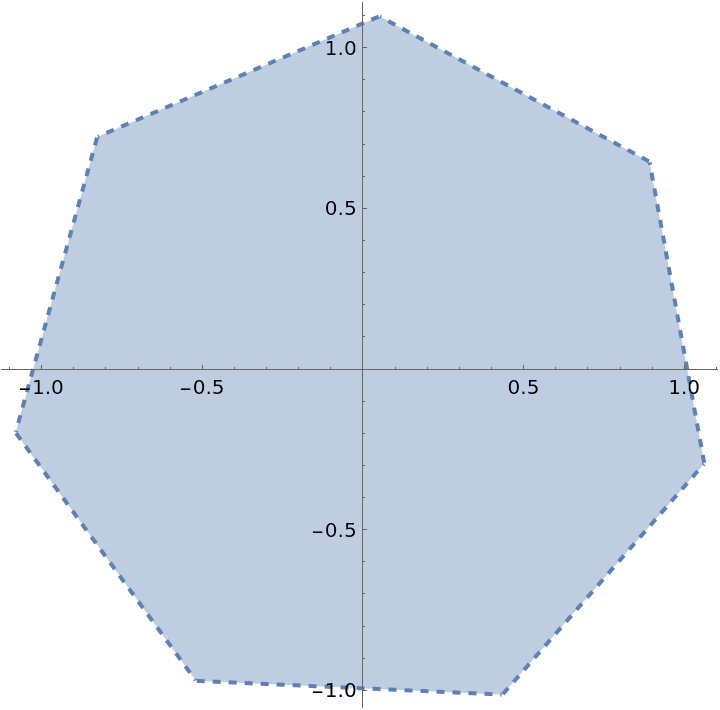With n very large (>100), one gets an approximation to a circle:

 In:=Out=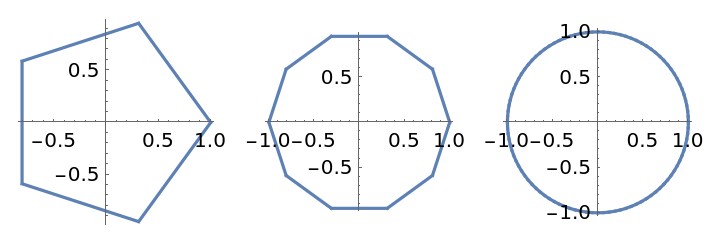### Neat Examples (2)

 In:=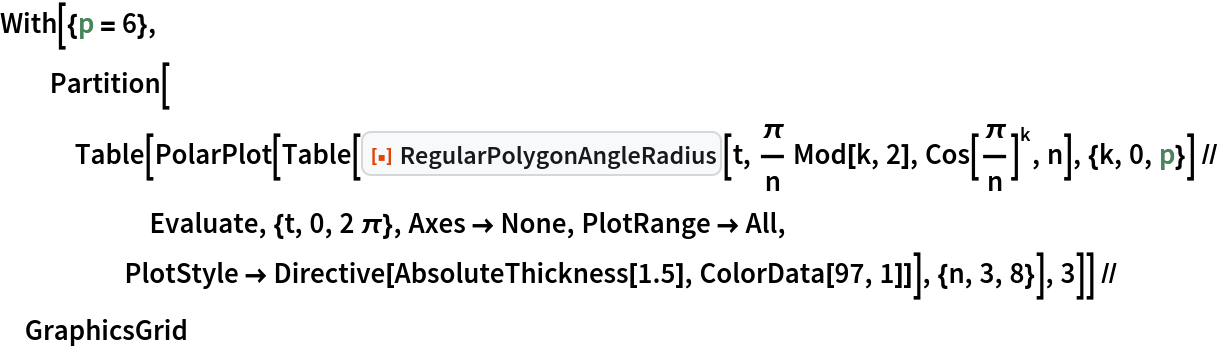Out=In:=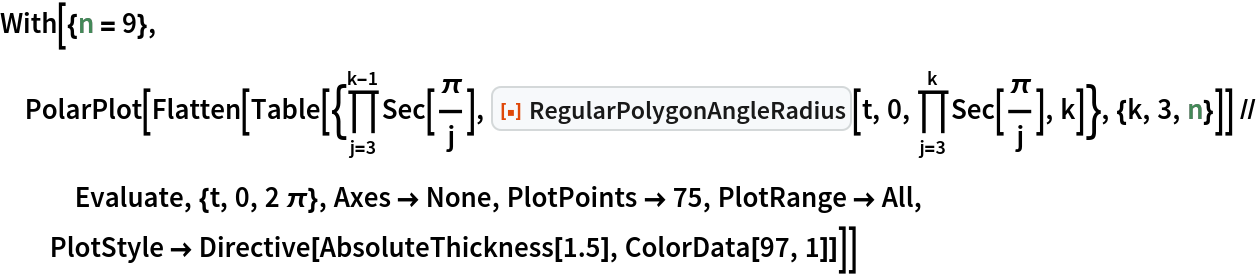Out=Erik Mahieu

## Version History

• 1.0.0 – 30 November 2021